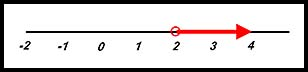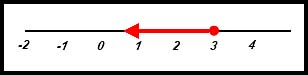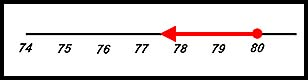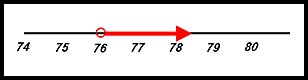Dr. J's Maths.com
Where the techniques of Maths
are explained in simple terms.

Algebra - Basic inequalities.
Test Yourself 2.

 x - 2 ≥ 4 a - 2 ≥ -3 2x ≤ 14.Writing the inequality. Write the inequality described in the following diagram:Write the inequality described in the following diagram:Write the inequality described in the following diagram:Write the inequality described in the following diagram: